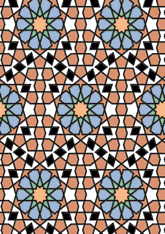# Eski Mosque, Edirnedata192/TUR0121

## Geometry

• The symmetry group of the tiling is 2*22 (cmm).
• All the internal angles of the constituent polygons are a multiple of 36°.
• Contains 10 regular two-pointed star polygons with vertex angle of 72°.
• Contains 6 regular pentagons.
• Contains two regular 10-pointed star polygons with vertex angle of 72°.
• There are 10 non-regular reflective tiles (including one kite).
• The tiling satisfies the interlace condition and has five finite interlaces and one infinite interlace with straight cross-overs.
• The tiling is edge-to-edge.
• As drawn, contains about 731 polygons.

## References

Publications referenced: